# YruRU Method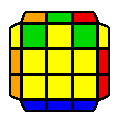##### (U') R U R' U R U2 R' U2 R' U' R U' R' U2 R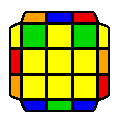##### (U2) R U' R' U2 R U R' U R' U' R U R U R' U' R' U R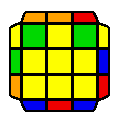##### R U2 R' U' R U' R2 U2 R U R' U R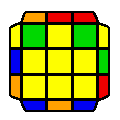##### (U2) R' U2 R U R' U R2 U2 R' U' R U' R'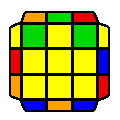##### (U') R' U' R2 U R2 U R2 U2 R' U R' U R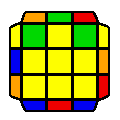##### (U') R U R2 U' R2 U' R2 U2 R U' R U' R'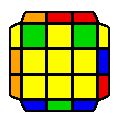##### R U2 R' U' R U' R' U R U R' U R U2 R'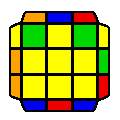##### R U' R U2 R U2 R' U R U R' U' R' U R'##### (U') R' U' R U' R' U2 R U R' U2 R U R' U R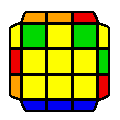##### (U') R U R' U R U2 R' U' R U2 R' U' R U' R'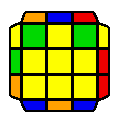##### (U2) R' U R' U2 R' U2 R U' R' U' R U R U' R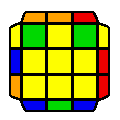##### (U2) R' U2 R U R' U R U' R' U' R U' R' U2 R

Average movecount: 14.5

Average double turns: 2.5

Algorithms: 12

If there is a 2x3 block, then it is a 2-twist case, which is simply 2 sune algorithms connected with a U move. If there is a 1x3 block (2 cases), then depending on the position of the block at the back, it again is 2 sune algorithms connected with a U move.

If there are no blocks (3 cases), then the 2x3 area may look like an H perm, or there may be a checker-board pattern extending to either right, or left.

If there are two blocks (2 cases) they will look like a Y perm, and their algorithms are simply 2 sune algorithms connected with a U move.

If there is a single block (4 cases), then the case can be decided based on the position of the block. If the block is at the back, then the algorithm is simply 2 sune algorithms connected with a U move.

Note, these rules are only to make learning of these cases simpler. For speed, a better recognition system is to be adopted.